Home
Hostname: page-component-768dbb666b-gx6zg Total loading time: 0.903 Render date: 2023-02-07T11:08:48.640Z Has data issue: true Feature Flags: { "useRatesEcommerce": false } hasContentIssue true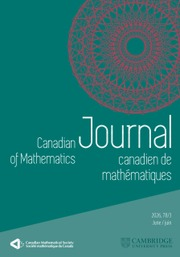Canadian Journal of Mathematics

# Steiner symmetry in the minimization of the first eigenvalue of a fractional eigenvalue problem with indefinite weight

Published online by Cambridge University Press:  14 April 2020

*

## Abstract

Let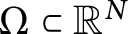$\Omega \subset \mathbb {R}^N$ ,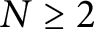$N\geq 2$ , be an open bounded connected set. We consider the fractional weighted eigenvalue problem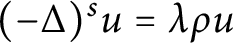$(-\Delta )^s u =\lambda \rho u$ in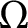$\Omega$ with homogeneous Dirichlet boundary condition, where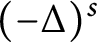$(-\Delta )^s$ ,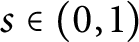$s\in (0,1)$ , is the fractional Laplacian operator,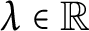$\lambda \in \mathbb {R}$ and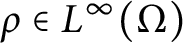$\rho \in L^\infty (\Omega )$ .

We study weak* continuity, convexity and Gâteaux differentiability of the map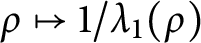$\rho \mapsto 1/\lambda _1(\rho )$ , where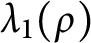$\lambda _1(\rho )$ is the first positive eigenvalue. Moreover, denoting by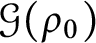$\mathcal {G}(\rho _0)$ the class of rearrangements of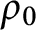$\rho _0$ , we prove the existence of a minimizer of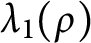$\lambda _1(\rho )$ when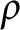$\rho$ varies on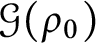$\mathcal {G}(\rho _0)$ . Finally, we show that, if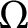$\Omega$ is Steiner symmetric, then every minimizer shares the same symmetry.

## MSC classification

Type
Article
Information
Canadian Journal of Mathematics , August 2021 , pp. 970 - 992

## Access options

Get access to the full version of this content by using one of the access options below. (Log in options will check for institutional or personal access. Content may require purchase if you do not have access.)

## References

Alvino, A., Trombetti, G. and Lions, P.L., On optimization problems with prescribed rearrangements. Nonlinear Anal. 13(1989), no. 2, 185220. https://doi.org/10.1016/0362-546x(89)90043-6 CrossRefGoogle Scholar
Anedda, C. and Cuccu, F., Steiner symmetry in the minimization of the first eigenvalue in problems involving the p-Laplacian. Proc. Amer. Math. Soc. 144(2016), 34313440. https://doi.org/10.1090/proc/12972 CrossRefGoogle Scholar
Anedda, C. and Cuccu, F., Symmetry breaking in the minimization of the second eigenvalue for composite membranes. Proc. Roy. Soc. Edinburgh Sect. A 145(2015), 111. https://doi.org/10.1017/s030821051300098x CrossRefGoogle Scholar
Baernstein, A. II, A unified approach to symmetrization. Sympos. Math., vol. XXXV, Cambridge Univ. Press, Cambridge (1994), 4791.Google Scholar
Berestycki, H., Le nombre de solutions de certains problèmes semi-linéaires elliptiques. J. Funct. Anal. 40(1981), 129. https://doi.org/10.1016/0022-1236(81)90069-0 CrossRefGoogle Scholar
Berestycki, H., Roquejoffre, J.-M. and Rossi, L., The periodic patch model for population dynamics with fractional diffusion. Discrete Contin. Dyn. Syst. Ser. S 4(1)(2011), 113. https://doi.org/10.3934/dcdss.2011.4.1 Google Scholar
Brezis, H., Functional analysis, Sobolev spaces and partial differential equations. Springer, New York (2011).CrossRefGoogle Scholar
Brock, F., Rearrangements and applications to symmetry problems in PDE. In: Handbook of differential equations, stationary partial differential equations, 4, Elsevier (2007), 160. https://doi.org/10.1016/s1874-5733(07)80004-0 Google Scholar
Bucur, C. and Valdinoci, E., Nonlocal diffusion and applications, 20. Springer, Bologna (2016).CrossRefGoogle Scholar
Burton, G.R., Rearrangements of functions, maximization of convex functionals and vortex rings . Math. Ann. 276(1987), 225253. https://doi.org/10.1007/bf01450739 CrossRefGoogle Scholar
Burton, G.R., Variational problems on classes of rearrangements and multiple configurations for steady vortices . Ann. Inst. H. Poincaré Anal. Non Linéaire 6(1989), no. 4, 295319. https://doi.org/10.1016/S0294-1449(16)30320-1 CrossRefGoogle Scholar
Cabré, X. and Sire, Y., Nonlinear equations for fractional Laplacians I: Regularity, maximum principles, and Hamiltonian estimates. Ann. Inst. Henri Poincaré (C) Nonlinear Analysis 31(2014), 2353. https://doi.org/10.1016/j.anihpc.2013.02.001 CrossRefGoogle Scholar
Caffarelli, L., Nonlocal diffusions, drifts and games. In: Nonlinear partial differential equations, Springer, Berlin Heidelberg (2012), 3752. https://doi.org/10.1007/978-3-642-25361-4_3 Google Scholar
Cantrell, R.S. and Cosner, C., Diffusive logistic equations with indefinite weights: population models in disrupted environments. Proc. R. Soc. Edinb. Sect A 112, 3-4(1989), 293318. https://doi.org/10.1017/s030821050001876x CrossRefGoogle Scholar
Cosner, C., Cuccu, F. and Porru, G., Optimization of the first eigenvalue of equations with indefinite weights . Adv. Nonlinear. Stud. 13(2013), 7995. https://doi.org/10.1515/ans-2013-0105 CrossRefGoogle Scholar
Cox, S.J. and McLaughlin, J.R., Extremal eigenvalue problems for composite membranes, I. Appl. Math. Optim. 22(1990), 153167. https://doi.org/10.1007/bf01447325 CrossRefGoogle Scholar
Cox, S.J. and McLaughlin, J.R., Extremal eigenvalue problems for composite membranes, II. Appl. Math. Optim. 22(1990), 169187. https://doi.org/10.1007/bf01447326 CrossRefGoogle Scholar
Day, P. W., Rearrangements of measurable functions. Ph.D. dissertation, California Institute of Technology (1970).Google Scholar
de Figueiredo, D.G., Positive solutions of semilinear elliptic problems. In: Dold, A. and Eckmann, B. (eds.), Differential equations, Lecture Notes in Mathematics 957, Springer, Berlin (1982), 3487. https://doi.org/10.1007/BFb0066233 Google Scholar
Di Nezza, E., Palatucci, G. and Valdinoci, E., Hitchhiker’s guide to the fractional Sobolev spaces. Bull. Sci. Math. 136(2012), 521573. https://doi.org/10.1016/j.bulsci.2011.12.004 CrossRefGoogle Scholar
Ekeland, I. and Témam, R., Convex analysis and variational problems. Classics in Applied Mathematics 28, SIAM (1999).CrossRefGoogle Scholar
Frassu, S. and Iannizzotto, A., Strict monotonicity and unique continuation for general non-local eigenvalue problems. Taiwanese J. Math. Advance Publication 24(2020), 681694. https://doi.org/10.11650/tjm/190709 Google Scholar
Hardy, G. H., Littlewood, J. E. and Pólya, G., Inequalities. 2nd ed., Cambridge University Press, Cambridge(1952, first published in 1934).Google Scholar
Henrot, A., Extremum problems for eigenvalues of elliptic operators. Frontiers in Mathematics, Birkhuser Verlag, Basel (2006).CrossRefGoogle Scholar
Iannizzotto, A. and Papageorgiou, N. S., Existence and multiplicity results for resonant fractional boundary value problems. Discrete Contin. Dyn. Syst. Ser. S 11(2018), 511532. https://doi.org/10.3934/dcdss.2018028 Google Scholar
Kawhol, B., Rearrangements and convexity of level sets in PDE. Lecture Notes in Mathematics, 1150, Springer, Berlin (1985).CrossRefGoogle Scholar
Lax, P.D., Functional analysis. Wiley, New York (2002).Google Scholar
Bisci, G. Molica, Radulescu, V.D. and Servadei, R., Variational methods for nonlocal fractional problems, 162. Cambridge University Press, Cambridge, UK (2016). https://doi.org/10.1017/cbo9781316282397 CrossRefGoogle Scholar
Olivares Contador, F., The Faber-Krahn inequality for the first eigenvalue of the fractional Dirichlet$p$ -Laplacian for triangles and quadrilateral. Pacific J. Math. 288(2017), 425434.https://doi.org/10.2140/pjm.2017.288.425 CrossRefGoogle Scholar
Pellacci, B. and Verzini, G., Best dispersal strategies in spatially heterogeneous environments: optimization of the principal eigenvalue for indefinite fractional Neumann problems. J. Math. Biol. 76(2018), 13571386. https://doi.org/10.1007/s00285-017-1180-z CrossRefGoogle ScholarPubMed
Ryff, J. V., Extreme points of some convex subsets of${L}^1\left(0,1\right)$ . Proc. Amer. Math. Soc. 18(1967), 10261034. https://doi.org/10.1090/s0002-9939-1967-0217586-3 Google Scholar
Servadei, R. and Valdinoci, E., Variational methods for non-local operators of elliptic type. Discrete Contin. Dyn. Syst. 33(2013), 21052137. https://doi.org/10.3934/dcds.2013.33.2105 CrossRefGoogle Scholar
Vázquez, J.L., Nonlinear diffusion with fractional Laplacian operators. In: Nonlinear partial differential equations, Abel. Symp. 7(2012), 271298. https://doi.org/10.1007/978-3-642-25361-4_15 CrossRefGoogle Scholar
2
Cited by

# Save article to Kindle

Note you can select to save to either the @free.kindle.com or @kindle.com variations. ‘@free.kindle.com’ emails are free but can only be saved to your device when it is connected to wi-fi. ‘@kindle.com’ emails can be delivered even when you are not connected to wi-fi, but note that service fees apply.

Find out more about the Kindle Personal Document Service.

Steiner symmetry in the minimization of the first eigenvalue of a fractional eigenvalue problem with indefinite weight
Available formats
×

# Save article to Dropbox

To save this article to your Dropbox account, please select one or more formats and confirm that you agree to abide by our usage policies. If this is the first time you used this feature, you will be asked to authorise Cambridge Core to connect with your Dropbox account. Find out more about saving content to Dropbox.

Steiner symmetry in the minimization of the first eigenvalue of a fractional eigenvalue problem with indefinite weight
Available formats
×

# Save article to Google Drive

To save this article to your Google Drive account, please select one or more formats and confirm that you agree to abide by our usage policies. If this is the first time you used this feature, you will be asked to authorise Cambridge Core to connect with your Google Drive account. Find out more about saving content to Google Drive.

Steiner symmetry in the minimization of the first eigenvalue of a fractional eigenvalue problem with indefinite weight
Available formats
×
×